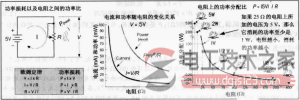`>`首页 > 电工元件 > 电阻器`>`电阻额定功率 正文

# 电阻额定功率重要性分析

## 电阻额定功率

V=IR=（0.001A）×（4700Ω）=4.7V；P=I2×R=（0.001A）2×（4700Ω）=0.0047W=4.7mW

R=V/I=（24V）/（0.05A）=480Ω；P=I×V=（0.05A）×（24V）=1.2WI=V/R=（3V）/（1000000Ω）=0.000003A=3μA；P=V2/R=（3V）2/（1000000Ω）=0.000009W=9μW

展开全文
`相关文章`